International
Tables for
Crystallography
Volume B
Reciprocal space
Edited by U. Shmueli

International Tables for Crystallography (2010). Vol. B, ch. 1.3, p. 45   | 1 | 2 |

## Section 1.3.2.6.9.3. Asymptotic distribution of eigenvalues of Toeplitz forms

G. Bricognea

aGlobal Phasing Ltd, Sheraton House, Suites 14–16, Castle Park, Cambridge CB3 0AX, England, and LURE, Bâtiment 209D, Université Paris-Sud, 91405 Orsay, France

#### 1.3.2.6.9.3. Asymptotic distribution of eigenvalues of Toeplitz forms

| top | pdf |

The eigenvalues of the Hermitian form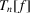are defined as thereal roots of the characteristic equation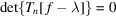. They will be denoted byIt is easily shown that iffor all x, thenfor all n and all. Asthese bounds, and the distribution of thewithin these bounds, can be made more precise by introducing two new notions.

 (i) Essential bounds: define ess inf f as the largest m such thatexcept for values of x forming a set of measure 0; and define ess sup f similarly. (ii) Equal distribution. For each n, consider two sets ofreal numbers:Assume that for eachand each n,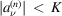and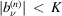with K independent ofand n. The setsandare said to be equally distributed inif, for any function F over,We may now state an important theorem of Szegö (1915, 1920). Let, and put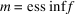,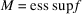. If m and M are finite, then for any continuous functiondefined in the interval [m, M] we have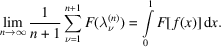In other words, the eigenvaluesof theand the valuesof f on a regular subdivision of ]0, 1[ are equally distributed.

Further investigations into the spectra of Toeplitz matrices may be found in papers by Hartman & Wintner (1950, 1954), Kac et al. (1953), Widom (1965), and in the notes by Hirschman & Hughes (1977).

### References

Hartman, P. & Wintner, A. (1950). On the spectra of Toeplitz's matrices. Am. J. Math. 72, 359–366.
Hartman, P. & Wintner, A. (1954). The spectra of Toeplitz's matrices. Am. J. Math. 76, 867–882.
Hirschman, I. I. Jr & Hughes, D. E. (1977). Extreme Eigenvalues of Toeplitz Operators. Lecture Notes in Mathematics, Vol. 618. Berlin: Springer-Verlag.
Kac, M., Murdock, W. L. & Szegö, G. (1953). On the eigenvalues of certain Hermitian forms. J. Rat. Mech. Anal. 2, 767–800.
Szegö, G. (1915). Ein Grenzwertsatz uber die Toeplitzschen Determinanten einer reellen positiven Funktion. Math. Ann. 76, 490–503.
Szegö, G. (1920). Beitrage zur Theorie der Toeplitzchen Formen (Erste Mitteilung). Math. Z. 6, 167–202.
Widom, H. (1965). Toeplitz matrices. In Studies in Real and Complex Analysis, edited by I. I. Hirschmann Jr, pp. 179–209. MAA Studies in Mathematics, Vol. 3. Englewood Cliffs: Prentice-Hall.